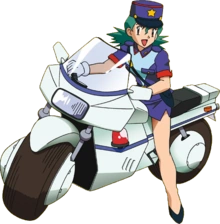Vehicle Builder: Engineer Feature
Prerequisites: Engineer, 5 Engineer Features
At-Will
Target: Items.
Effect: You may build a vehicle using the following price guidelines. Your GM will determine how quickly you make the vehicle. Name the vehicle whatever you’d like. Build it to look like whatever you’d like.

# Pricing Guidelines

The following are advanced pricing guidelines for building vehicles. They allow for realistic costs and the possibility of build vehicles of impressive size.

## Material Coverage/Weight and Shell Cost

The most important stat. This determines how big the craft is, and, based on the size, the cost of the vehicle gradually increases. In short, the bigger the vehicle, the heavier it is.

Material Coverage is determined by how many square of floor and, if applicable, ceiling space the vehicle has. If a square has only, say, a engine in it, it still counts towards coverage.

Passengers
A Vehicle can support as many passengers as it has space to do so.

Walls
The walls of a vehicle are for vehicles such as cars, planes, and trucks. They provide protection for people inside of them from the environment, as well as other things.
For sanity sake, a builder is charged only for wall squares past 1 meters in height.

(1)
\begin{equation} Wall Squares (min:0) = (Wall Segment Height -1)*Number of Wall Segments \end{equation}
• Common Wall Heights
• Bike = 0 meter
• Motorcycle = 0 meter
• Car = 1 meter
• Truck = 2 meter

Materials
A vehicle can be built out of lightweight or heavy materials, and even have later additions to it built out of such material. The exact ramifications of which, including cost, can be up to the GM.

Material Weight Modifier Cost Modifier Notes
Steel 1 1 -
Plastic ? ? -
Glass ? ? -
(2)
\begin{equation} Coverage = Ceiling squares + Floor Squares + Wall Squares \end{equation}
(3)
\begin{equation} Vehicle Weight = Sum(Coverage * Material Weight Modifier) \end{equation}
(4)
\begin{equation} Shell Cost = Sum(Coverage * Material Cost Modifier) \end{equation}

## Power Source

Depending on the size of vehicle, the power source should vary. The larger the vehicle, the larger the power source.
Each Power source has its own cost. It could be possible to mix and match the power sources to cover the needed area, and possibly some surplus in case one fails or is destroyed.

The Size of the engine can vary.

Engine Source Engine Cost Modifier Notes
Pokemon/Man Engine Free 1
Gas Engine 30 1 -
Electric Engine 78 -

Engine Capacity
Engine Capacity is determined by how much vehicle weight it can push.
An Engine can push that much vehicle weight, plus an additional 100% of the vehicles apparent weight.

Note: For now, lets assume a engine can provide enough power to carry 200% of the vehicle weight it was designed for. Of course, loading all the way up to 200% can possibly over-strain the engine.

(5)
\begin{equation} Engine Capacity = Vehicle Weight \end{equation}
(6)
\begin{equation} Engine Cost = Engine Capacity * Engine Cost Modifier \end{equation}

1 How much engine power pokemon and humans is related the power/strength and endurance/constitution of each Pokemon/Trainer powering the engine. The Engine Capacity in this case is $( Sum(Max Carrying Capacity of Each Involved) ) / 2$

## Movement Modes

In order to gain a movement capability, you need to put purchase at least 2 points of it.
The speed also will likely have to relay on square coverage, as the bigger the craft, the bigger/more jets/wheels/propellors.
Each capability is separated to show that, while a vehicle may be fast on water, it could be slow on land.

Movement Capability Movement Cost Modifier Notes
Overland 100 -
Surface 265 -
Underwater 300 -
Burrow 775 -
Sky 1675 -
(7)
\begin{equation} Movement Capability Cost=Movement Cost Modifier*Capability Value*Weight \end{equation}

## HP

HP Cost = 1 per HP, maximum 4500

## Extra Features

It is possible to add extra features to your vehicle. The cost and mechanics of which is up to the GM. Here are some suggestions.

• Air Supply (for under water and burrow) per minute
• Air Compressor (for refilling air supply)
• Armor, to reduce damage.
• Heating and Cooling
• Communications Array
• Solar Array, to recharge electricity.
• Kitchen
• Bunks
• Speed Booster
• Weapon

## Improving the Vehicle

With this system, it is possible to improve a vehicle. The exact process can vary to games, but a possible way is to allow the Engineer is to reduce costs from one part of the vehicle in order to improve others, possibly leaving leftover materials.

# Examples of VehiclesBike: 1 square meter, man powered. Land 15 (Thanks Gents)
Original Base Cost: 1000+2340=3340
New Base Cost: 1500+1000=2500
Special: 50 km/h overland. Jump 1Motor Bike: 2 square meter, gas powered. Land 25
Original Base Cost: 400+2340+1000=3740
New Base Cost: 2000+5000+60=7060
Special: 200 km/h overland.ZZ Flyer: 1 square meter. Electric Power. Sky 10.
Old Base Cost: 16750+2340+250+780=20120
New Base Cost: 16828ZERO ONE: 3 square meters (3 people). 5 meters land, 6 meters surface, 4 meters air. Electric Powered
Old Cost: 16750+2650+1000+250+9840+780=31270
New Cost: 3000+234+1500+4770+20100=29604
Special: Capable of switching between modes as a trainer action.
Optional Feature: Dash Boost. Increase speed capabilities by 4 for a minute. Cost 2000.

Normal Hot Air Baloon
Sky 1, power gas. 4 square meters.
Old price: 16750+400+9840=26990
New Price: 4000+160+6700=10860
Special: Speed in the sky can vary depending on the wind.

page revision: 10, last edited: 26 Oct 2011 16:38